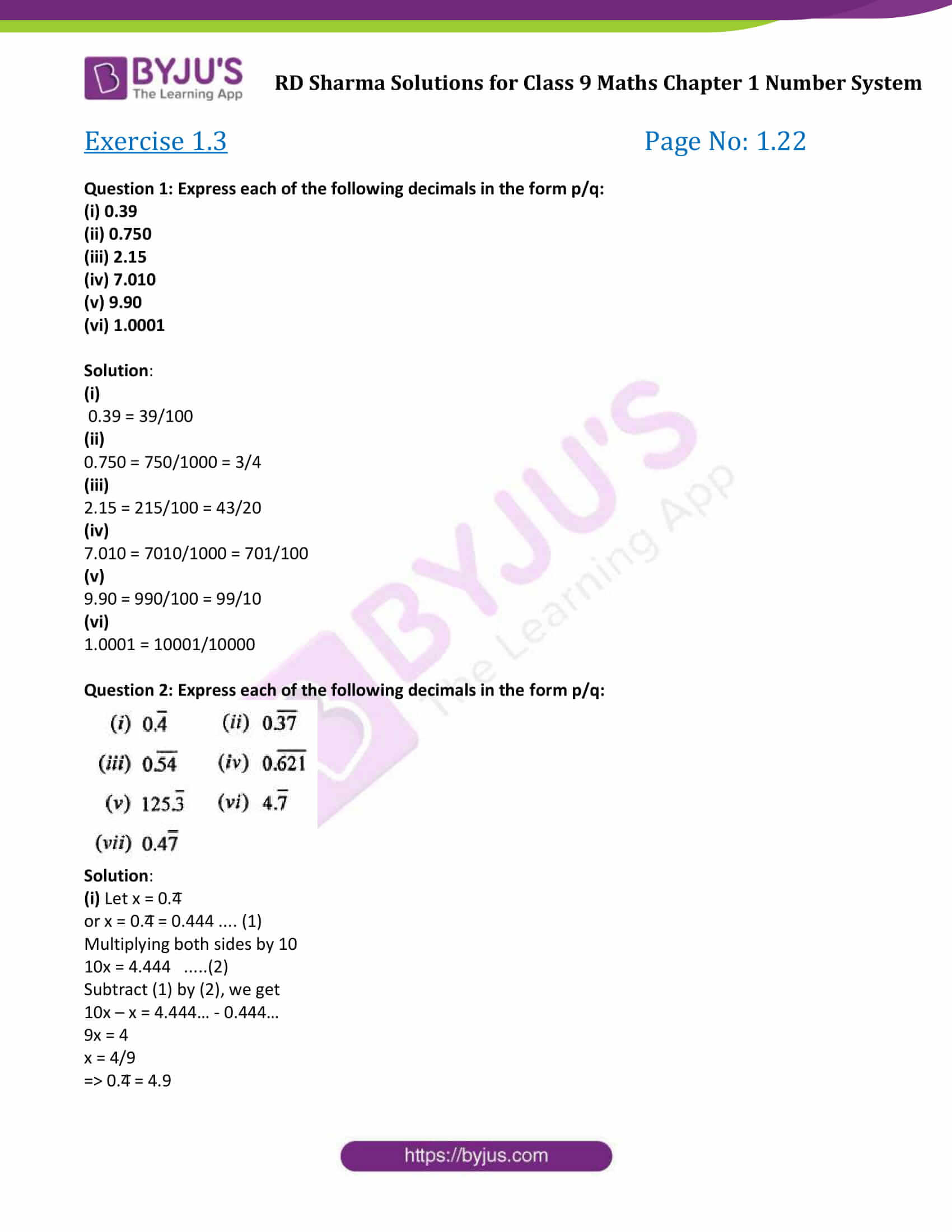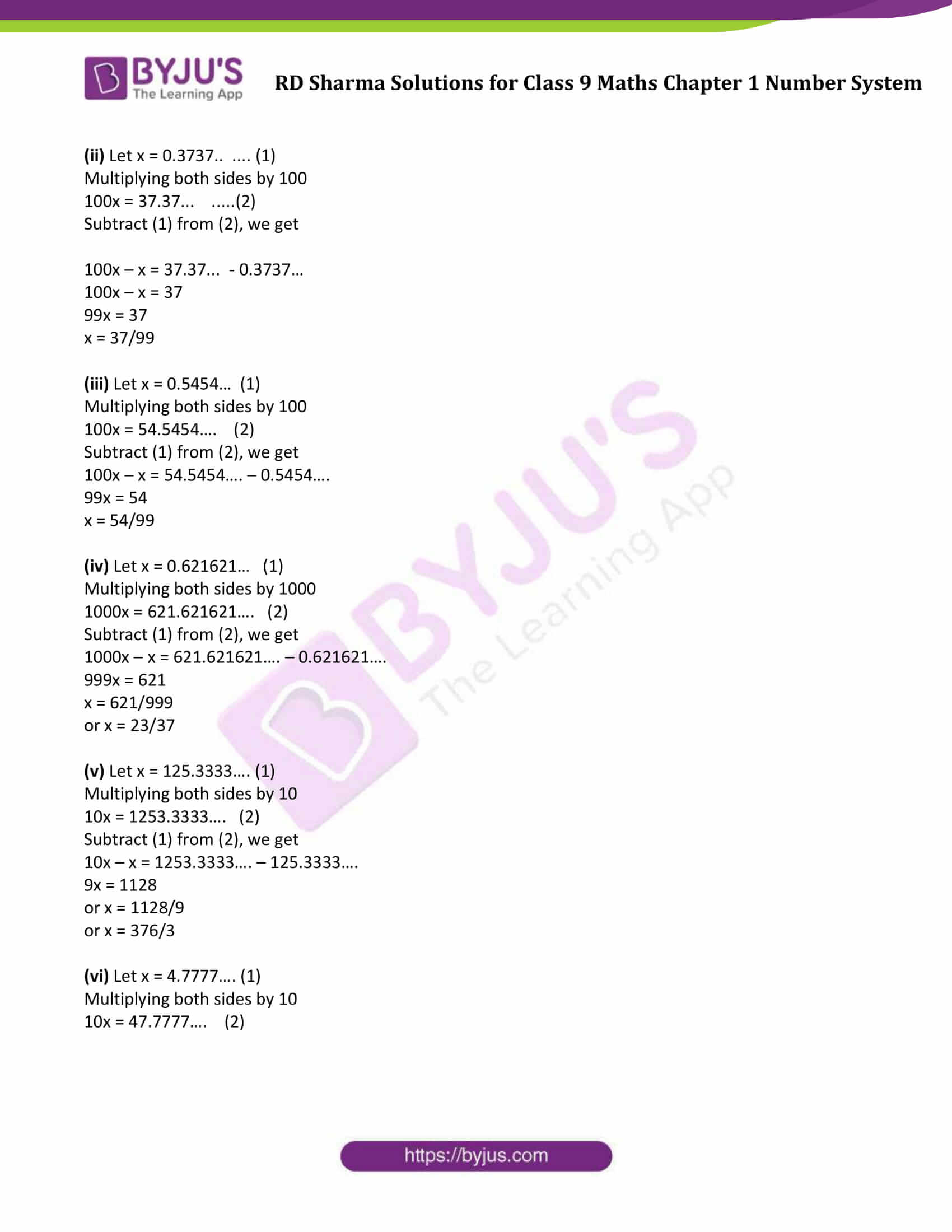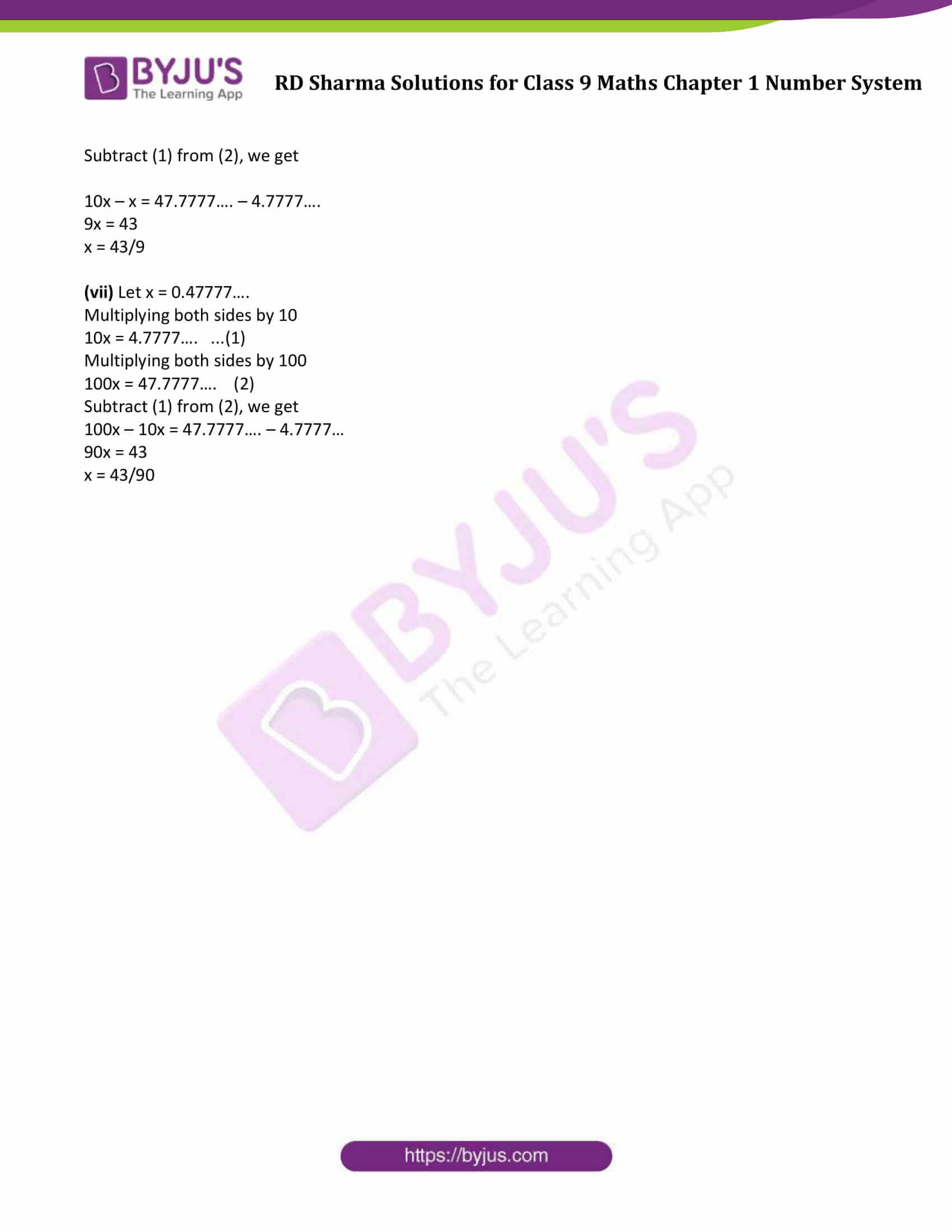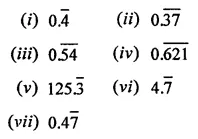# RD Sharma Solutions Class 9 Maths Chapter 1 Number System Exercise 1.3

RD Sharma Class 9 Mathematics Chapter 1 Exercise 1.3 Number System is provided here. RD Sharma Solutions Class 9 chapter 1 textbook questions will help students to understand the concepts and to practice higher-level questions related to the number system. This exercise based on the conversion of decimal numbers into rational numbers. Click on the link below to download free PDF of class 9 chapter 1.

## Download PDF of RD Sharma Solutions for Class 9 Maths Chapter 1 Number System Exercise 1.3### Access Answers to Maths RD Sharma Solutions for Class 9 Chapter 1 Number System Exercise 1.3

Question 1: Express each of the following decimals in the form p/q:

(i) 0.39

(ii) 0.750

(iii) 2.15

(iv) 7.010

(v) 9.90

(vi) 1.0001

Solution:

(i)

0.39 = 39/100

(ii)

0.750 = 750/1000 = 3/4

(iii)

2.15 = 215/100 = 43/20

(iv)

7.010 = 7010/1000 = 701/100

(v)

9.90 = 990/100 = 99/10

(vi)

1.0001 = 10001/10000

Question 2: Express each of the following decimals in the form p/q:Solution:

(i) Let x = 0.4̅

or x = 0.4̅ = 0.444 …. (1)

Multiplying both sides by 10

10x = 4.444 …..(2)

Subtract (1) by (2), we get

10x – x = 4.444… – 0.444…

9x = 4

x = 4/9

=> 0.4̅ = 4.9

(ii) Let x = 0.3737.. …. (1)

Multiplying both sides by 100

100x = 37.37… …..(2)

Subtract (1) from (2), we get

100x – x = 37.37… – 0.3737…

100x – x = 37

99x = 37

x = 37/99

(iii) Let x = 0.5454… (1)

Multiplying both sides by 100

100x = 54.5454…. (2)

Subtract (1) from (2), we get

100x – x = 54.5454…. – 0.5454….

99x = 54

x = 54/99

(iv) Let x = 0.621621… (1)

Multiplying both sides by 1000

1000x = 621.621621…. (2)

Subtract (1) from (2), we get

1000x – x = 621.621621…. – 0.621621….

999x = 621

x = 621/999

or x = 23/37

(v) Let x = 125.3333…. (1)

Multiplying both sides by 10

10x = 1253.3333…. (2)

Subtract (1) from (2), we get

10x – x = 1253.3333…. – 125.3333….

9x = 1128

or x = 1128/9

or x = 376/3

(vi) Let x = 4.7777…. (1)

Multiplying both sides by 10

10x = 47.7777…. (2)

Subtract (1) from (2), we get

10x – x = 47.7777…. – 4.7777….

9x = 43

x = 43/9

(vii) Let x = 0.47777….

Multiplying both sides by 10

10x = 4.7777…. …(1)

Multiplying both sides by 100

100x = 47.7777…. (2)

Subtract (1) from (2), we get

100x – 10x = 47.7777…. – 4.7777…

90x = 43

x = 43/90

## RD Sharma Solutions for Class 9 Maths Chapter 1 Number System Exercise 1.3

RD Sharma Solutions Class 9 Maths Chapter 1 Number System Exercise 1.3 is based on following topics and subtopics:

• Conversion of decimal numbers into rational numbers of the form m/n
• Conversion of terminating decimal number to the form p/q
• Conversion of a pure recurring decimal to the form p/q
• Conversion of a mixed recurring decimal to the form p/q